# Arduino PID Temperature Controller using MAX6675 K-Thermocouple to Digital Converter IC

Published  November 1, 2021   2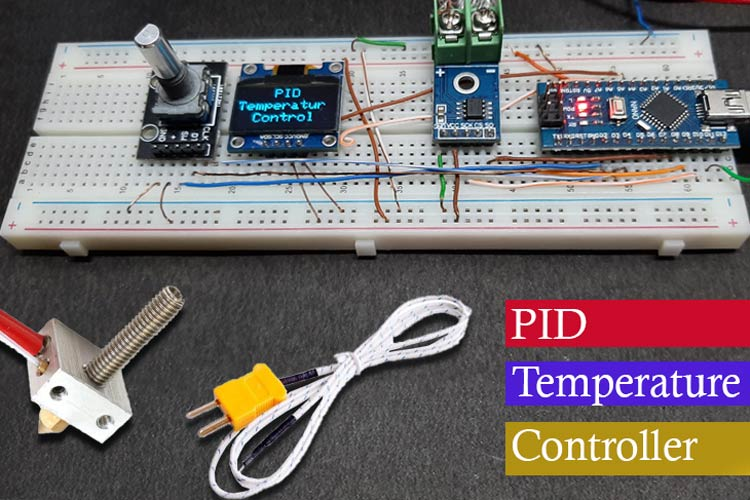In this article we decided to make a PID Controller based Heater that can be used to control the temperature of the hot end of the 3D printer or by slightly modifying the device it can control the temperature of a DC Soldering Iron very efficiently, and a little more tweak and you can control a TRIAC and that can control the RPM of an AC motor or an AC heating element the possibilities are limitless with PID control.

In one of our previous articles, we made a PID controller based self-balancing robot and an Encoder Motor Control with PID you can check those out if you want to learn more about the topic.

### What is a Temperature PID Controller ?

As the name suggests a temperature PID controller deals with temperature, PID temperature control is a closed-loop control algorithm that improves the accuracy of the process. The PID temperature control works using a mathematical formula to calculate the difference between the current temperature and setpoint. And then it tries to deliver the required power to ensure the target temperature remains constant, this not only reduces environmental impact but it also reduces overshoots that can be found in the traditional on-off control mechanism.

### How does a Temperature PID Controller work?

As in any PID control first, we need to be aware of the output or what we want the controller to do, for this project we want to maintain a certain temperature of the heating element (we will set that temperature with the help of the rotary encoder) so to maintain the temperature we need to read out of the temperature, for that we are using a K-type thermocouple, in conjunction with MAX6675 Cold-Junction-Compensated K-Thermocouple to Digital Converter IC that can measure hundreds of degree Celsius without any issues. And the temperature readout from the thermocouple acts as feedback. Now as we have set the temperature we want to achieve and we have a real-time readout of the temperature value the controller can calculate the error value and with the help of proportional integral and derivative control the system can achieve its target, for this project we will control a PWM signal with the calculated output value. That is how a Temperature Based PID controller works.

### MAX6675 K-Thermocouple IC Working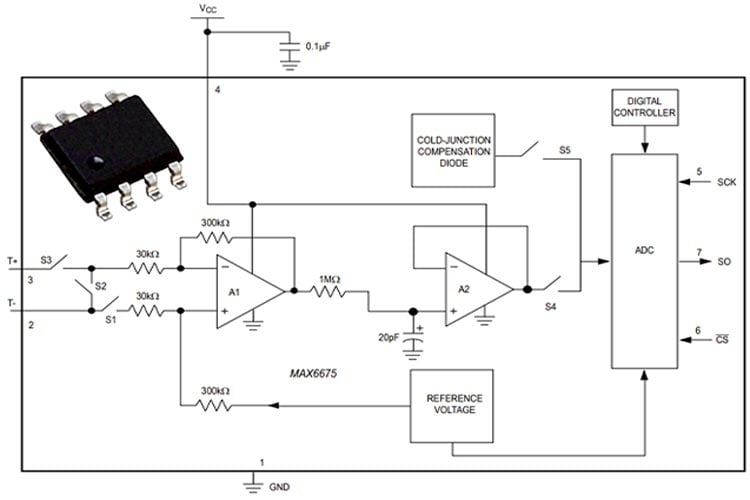The function of the thermocouple is to sense a difference in temperature between two ends of the thermocouple wires. The thermocouple’s hot junction can be read from 0°C to +1023.75°C. The cold end (ambient temperature of the board on which the MAX6675 is mounted) can only range from -20°C to +85°C. While the temperature at the cold end fluctuates, the MAX6675 continues to accurately sense the temperature difference at the opposite end. The MAX6675 senses and corrects for the changes in the ambient temperature with cold-junction compensation. The device converts the ambient temperature reading into a voltage using a temperature-sensing diode. To make the actual thermocouple temperature measurement, the MAX6675 measures the voltage from the thermocouple’s output and the sensing diode. The device’s internal circuitry passes the diode’s voltage (sensing ambient temperature) and thermocouple voltage (sensing remote temperature minus ambient temperature) to the conversion function stored in the ADC to calculate the thermocouple’s hot-junction temperature. Optimal performance from the MAX6675 is achieved when the thermocouple cold junction and the MAX6675 are at the same temperature. The MAX6675 includes signal-conditioning hardware to convert the thermocouple’s signal into a voltage compatible with the input channels of the ADC. The T+ and T inputs connect to internal circuitry that reduces the introduction of noise errors from the thermocouple wires. More information can be found on the Datasheet of the MAX6675 IC.

### Components Required to build a PID Enabled Temperature Controller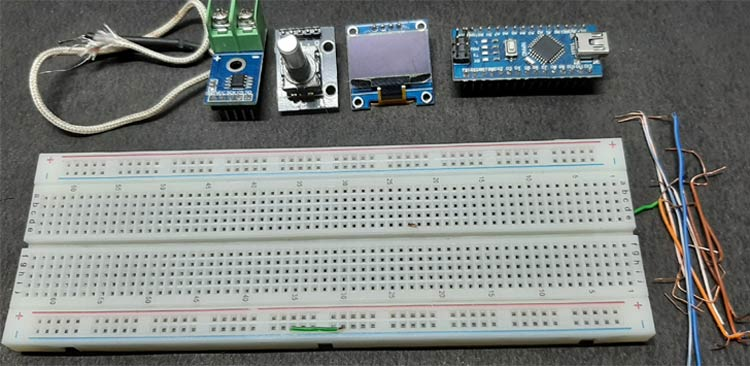The components required to build the MAX6675 based PID Controlled Heater are listed below, we designed this circuit with very generic components, which makes the replication process very easy.

• Arduino Nano - 1
• 128 X 64 OLED Display - 1
• Generic Rotary Encoder - 1
• MAX6675 Module - 1
• K-type Thermocouple - 1
• Jumper Wires - 1

### PID Enabled Temperature Controller Circuit Diagram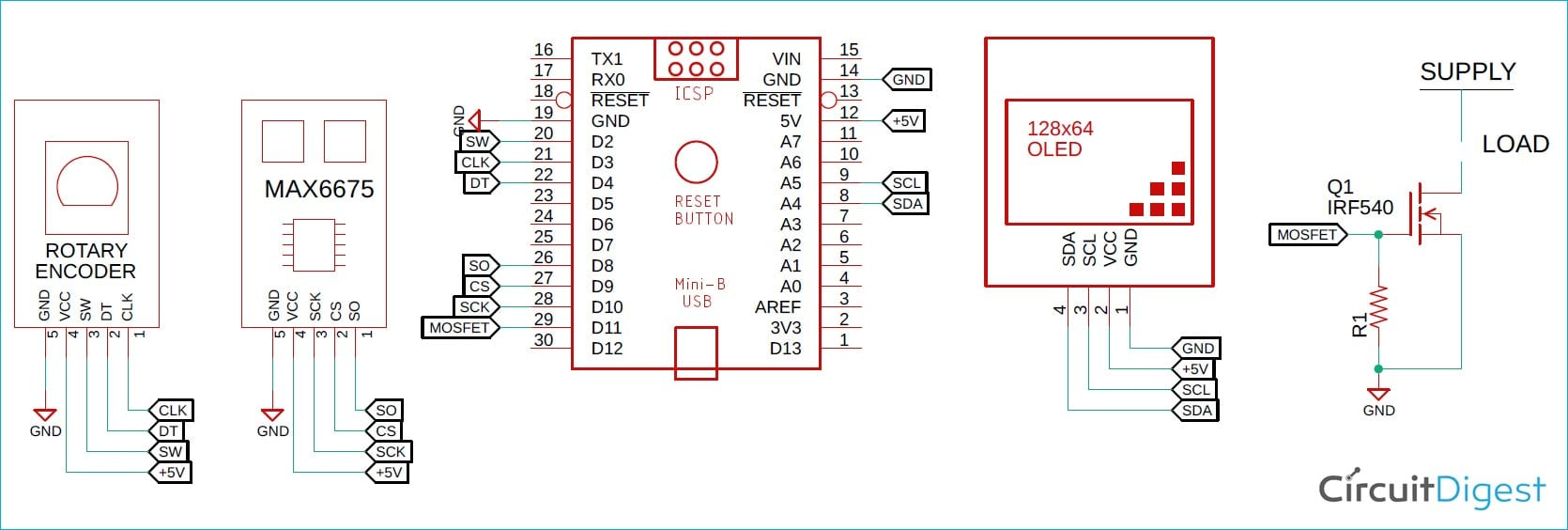In this project, we use the MAX6675 K-type Thermocouple sensor to read the temperature data from the thermocouple, and in this section, we will explain all the details with the help of the schematic. Let me give you a brief overview of what is happening with this circuit. The MAX6675 is a Cold-Junction-Compensated K- Thermocouple to Digital Converter module and it connects to Arduino according to the schematic. The power is provided to the circuit with the help of a +5V supply of the Arduino. Also to set the temperature and change the modes we are using a generic Rotary encoder if you want to learn more about how rotary encoder works we have a detailed article on how to interface rotary encoder with Arduino. Next, we have the 128X64 OLED display the display shows the temperature data and it also shows the set temperature. If you also want to learn more about OLED display and its interfacing strategies with Arduino there is also a dedicated article on it. As always, we are using Arduino as the brains of the project. By pressing the button on the rotary encoder, we can switch in between two modes one is to set the temperature and another one to monitor the trout from the thermocouple. Other than that, the circuit stays pretty simple.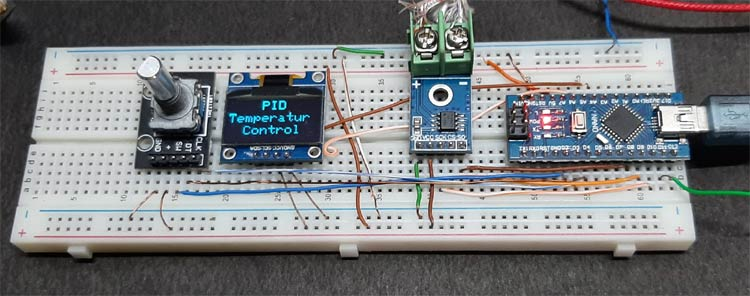### MAX6675 based PID Enabled Temperature Controller Arduino Code

The complete code used in this project can be found at the bottom of this page. After adding the required header files and source files, you should be able to directly compile the Arduino code without any errors. You can download the PID controller library, MAX6675 Library, AAdafruit_SSD1306 Library from the link given below, or else you can use the board manager method to install the library.

A simple explanation of the code is given as comments, and in this section, we will go a little more in-depth about it. First, we start by including all the required libraries, after that, we define all the necessary pins that are required to read the encoder, drive the OLED and MAX6675 Thermocouple temperature sensor. Once that is done, we define all the values for the Kp, Ki, and Kd, and include all the required variables.

Next, we have defined the __Kp, __Ki, and __Kd values for our code. These three constants are responsible for setting up the output response for our code. Please do note that for this project, I have used the trial-and-error method to set the constants, but you can calculate the values if that is something necessary for your project.

```/*In this section we have defined the gain values for the
* proportional, integral, and derivative controller I have set
* the gain values with the help of trial and error methods.
*/
#define __Kp 30 // Proportional constant
#define __Ki 0.7 // Integral Constant
#define __Kd 200 // Derivative Constant```

Next, we declare all the required variables and we create three instances one for PID one for the OLED, and the final one for the thermocouple. Variable clockPin and clockPinStatedebounce, and encoder_btn_count all four used to read data from the encoder the temperature_value_c holds the temperature readout from the thermocouple, finally the encoder_btn_count holds how many times the button of the encode is pressed.

```#include <SPI.h>
#include <Wire.h>
#include <PIDController.h>
#include "max6675.h"
// Define Rotary Encoder Pins
#define CLK_PIN 3
#define DATA_PIN 4
#define SW_PIN 2
// MAX6675 Pins
#define thermoDO 8
#define thermoCS 9
#define thermoCLINE 10
// Mosfet Pin
#define mosfet_pin 11
// Serial Enable
#define __DEBUG__
#define SCREEN_WIDTH 128 // OLED display width, in pixels
#define SCREEN_HEIGHT 64 // OLED display height, in pixels
#define OLED_RESET -1 // Reset pin # (or -1 if sharing Arduino reset pin)
int clockPin; // Placeholder por pin status used by the rotary encoder
int clockPinState; // Placeholder por pin status used by the rotary encoder
int set_temperature = 1; // This set_temperature value will increas or decreas if when the rotarty encoder is turned
float temperature_value_c = 0.0; // stores temperature value
long debounce = 0; // Debounce delay
int encoder_btn_count = 0; // used to check encoder button press
MAX6675 thermocouple(thermoCLK, thermoCS, thermoDO); // Create an instance for the MAX6675 Sensor Called "thermocouple"
Adafruit_SSD1306 display(SCREEN_WIDTH, SCREEN_HEIGHT, &Wire, OLED_RESET);// Create an instance for the SSD1306 128X64 OLED "display"
PIDController pid; // Create an instance of the PID controller class, called "PID"```

Next, we have the setup function. In the setup function, first we enable the serial monitor for debugging but the Serial.begin() method is wrapped with a #ifdef and #endif statement so that we can easily disable the serial monitor once our code is complete.

```void setup() {
#ifdef __DEBUG__
Serial.begin(9600);
#endif```

Next, we have set all our required pins as input and output as required for our project to work.

```pinMode(mosfet_pin, OUTPUT); // MOSFET output PIN
pinMode(CLK_PIN, INPUT); // Encoer Clock Pin
pinMode(DATA_PIN, INPUT); //Encoder Data Pin
pinMode(SW_PIN, INPUT_PULLUP);// Encoder SW Pin```

Next, we initialize the PID controller by calling the begin() method of the PID instance. Next, we add setpoint using the setpoint() method the PID algorithm will try to reach the value by controlling the output. Next, we call the tune() method, this is where we put all the values for __Kp, __Ki, and __Kd. Finally, we set a limit for the PID controller this limiter will allow the calculated output to range within the limit this ensures the calculated output cannot go beyond a certain level that is 255 for our PWM value.

```pid.begin();          // initialize the PID instance
pid.setpoint(150);    // The "goal" the PID controller tries to "reach"
pid.tune(__Kp, __Ki,__Kd);    // Tune the PID, arguments: kP, kI, kD
pid.limit(0, 255);    // Limit the PID output between 0 and 255, this is important to get rid of integral windup!```

Next, we check if we have the display available or not, if the display is available the code will continue, and if the display is not available it will print an error.

```if (!display.begin(SSD1306_SWITCHCAPVCC, 0x3C)) {
#ifdef __DEBUG__
Serial.println(F("SSD1306 allocation failed"));
#endif
for (;;); // Don't proceed, loop forever
}```

Next, we set up the display and we start off by rotating the display and sowing a splash screen on the display, next we clear the display, setTextSize, and setTextColour to white. Next, we set the cursor in a different location and print PID Temperatur Control in the display and we finish off with a delay of 2 seconds.

```display.setRotation(2); //Rotate the Display
display.display(); //Show initial display buffer contents on the screen -- the library initializes this with an Adafruit splash screen.
display.clearDisplay(); // Cleear the Display
display.setTextSize(2); // Set text Size
display.setTextColor(WHITE); // set LCD Colour
display.setCursor(48, 0); // Set Cursor Position
display.println("PID"); // Print the this Text
display.setCursor(0, 20);  // Set Cursor Position
display.println("Temperatur"); // Print the this Text
display.setCursor(22, 40); // Set Cursor Position
display.println("Control"); // Print the this Text
display.display(); // Update the Display
delay(2000); // Delay of 200 ms```

Next, we have the set_temp() function. In this function, we set the temperature of the heater, and check the button status, if the status is two, then we first clear the display set the text size, and print the set temperature on the display. Next, we have the read_encoder() function as the name suggests we read the encoder and check the encoder is rotating clockwise or anti-clockwise, if the encoder is rotating clockwise we increment the counter if the encoder is rotating counter-clockwise we decrement the counter. Finally, we also check the encoder button status with the encoder button we can switch between temperature set mode and monitor mode.

```void set_temp(){
if (encoder_btn_count == 2) // check if the button is pressed twice and its in temperature set mode.
{
display.clearDisplay(); // clear the display
display.setTextSize(2); // Set text Size
display.setCursor(16, 0); // set the diplay cursor
display.print("Set Temp."); // Print Set Temp. on the display
display.setCursor(45, 25); // set the cursor
display.print(set_temperature);// print the set temperature value on the display
display.display(); // Update the Display
}
}

void read_encoder() // In this function we read the encoder data and increment the counter if it is rotating clockwise and decrement the counter if it's rotating counterclockwise
{
clockPin = digitalRead(CLK_PIN); // we read the clock pin of the rotary encoder
if (clockPin != clockPinState && clockPin == 1) { // if this condition is true then the encoder is rotaing counter clockwise and we decremetn the counter
if (digitalRead(DATA_PIN) != clockPin) set_temperature = set_temperature - 3;  // decrmetn the counter.
else set_temperature = set_temperature + 3; // Encoder is rotating CW so increment
if (set_temperature < 1 )set_temperature = 1; // if the counter value is less than 1 the set it back to 1
if (set_temperature > 150 ) set_temperature = 150; //if the counter value is grater than 150 then set it back to 150
#ifdef __DEBUG__
Serial.println(set_temperature); // print the set temperature value on the serial monitor window
#endif
}
clockPinState = clockPin; // Remember last CLK_PIN state
if ( digitalRead(SW_PIN) == LOW)   //If we detect LOW signal, button is pressed
{
if ( millis() - debounce > 80) { //debounce delay
encoder_btn_count++; // Increment the values
if (encoder_btn_count > 2) encoder_btn_count = 1;
#ifdef __DEBUG__
Serial.println(encoder_btn_count);
#endif
}
debounce = millis(); // update the time variable
}
}```

Finally, we have our loop function. In the loop function we call our read_encoder() and set_temp() function, these functions will be called continuously, and in the loop, we also check the button mode, and if it’s set to one we read the temperature from the thermocouple and put it through the compute method of the PID instance. Once the calculation is done we directly put the calculated values in the analog write function that outputs a PWM signal. Once everything is done, we just update the display and that marks the end of the code section.

```void loop()
{
set_temp(); // Call the Set Temperature Function
if (encoder_btn_count == 1) // check if the button is pressed and it's in Free Running mode -- in this mode, the Arduino continuously updates the screen and adjusts the PWM output according to the temperature.
{
int output = pid.compute(temperature_value_c);    // Let the PID compute the value, returns the optimal output
analogWrite(mosfet_pin, output);           // Write the output to the output pin
pid.setpoint(set_temperature); // Use the setpoint methode of the PID library to
display.clearDisplay(); // Clear the display
display.setTextSize(2); // Set text Size
display.setCursor(16, 0); // Set the Display Cursor
display.print("Cur Temp."); //Print to the Display
display.setCursor(45, 25);// Set the Display Cursor
display.print(temperature_value_c); // Print the Temperature value to the display in celcius
display.display(); // Update the Display
#ifdef __DEBUG__
Serial.print(temperature_value_c); // Print the Temperature value in *C on serial monitor
Serial.print(" "); // Print an Empty Space
Serial.println(output); // Print the Calculate Output value in the serial monitor.
#endif
delay(200); // Wait 200ms to update the OLED dispaly.
}
}```

### PID Enabled Temperature Controller Testing

To test the circuit the following setup is used, as you can see, I have connected my Meco 450B+ Multimeter to display the duty cycle of the output PWM signal which is from pin11 of the Arduino. And as a heater, I have used a 3D printer extruder, that contains a 12V heating element, to power the Arduino I have used the 5V power supply of my laptop and to power the heating element I have used an external 12V power source.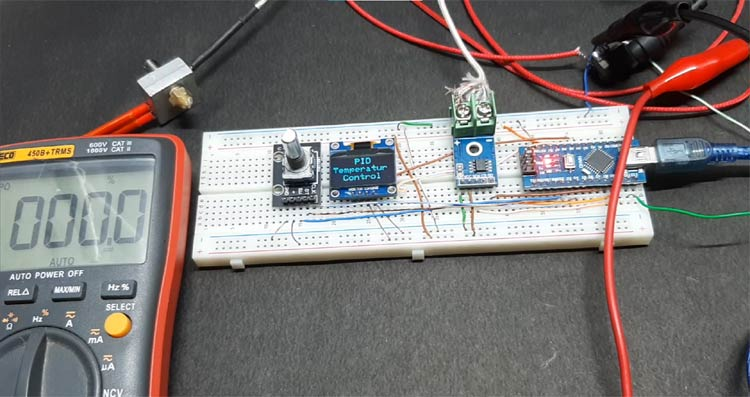Now, to set the temperature you need to press the button of the rotary encoder, which set the setpoint or the target temperature of the PID algorithm once set press the button another time to make the changes permanent and the heater block starts heating and you can see the duty cycle also increases, I have set the temperature to 64*C.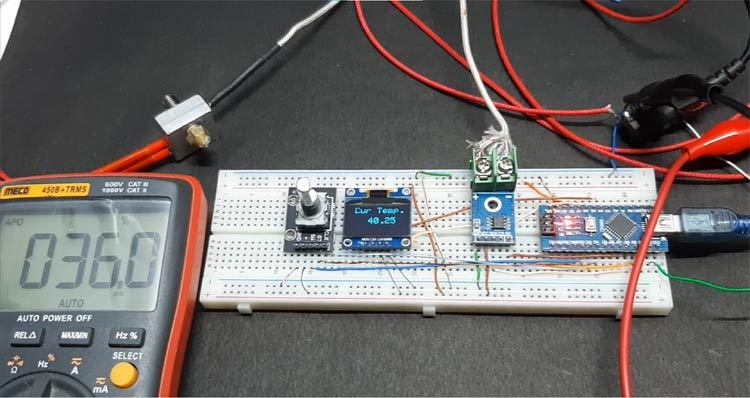Once the desired temperature is reached the PWM duty cycle is reduced and you can observe a certain spike in the duty cycle when the controller wants to compensate for the error and increase the temperature.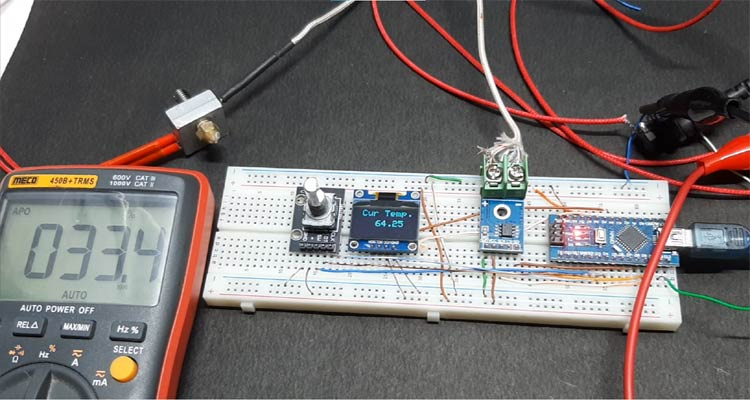This marks the end of the tutorial. I hope you liked the article and learned something new. If you have any questions regarding the article, you can leave them in the comment section below or you can use our Electronics Forum. You can also check out the video at the bottom.

Code

PID_temperature_Control.ino

```#include <SPI.h>
#include <Wire.h>
#include <PIDController.h>
#include "max6675.h"
// Define Rotary Encoder Pins
#define CLK_PIN 3
#define DATA_PIN 4
#define SW_PIN 2
// MAX6675 Pins
#define thermoDO  8
#define thermoCS  9
#define thermoCLK  10
// Mosfet Pin
#define mosfet_pin 11
// Serial Enable
#define __DEBUG__
#define SCREEN_WIDTH 128 // OLED display width, in pixels
#define SCREEN_HEIGHT 64 // OLED display height, in pixels
#define OLED_RESET     -1 // Reset pin # (or -1 if sharing Arduino reset pin)
/*In this section we have defined the gain values for the
* proportional, integral, and derivative controller I have set
* the gain values with the help of trial and error methods.
*/
#define __Kp 30 // Proportional constant
#define __Ki 0.7 // Integral Constant
#define __Kd 200 // Derivative Constant
int clockPin; // Placeholder por pin status used by the rotary encoder
int clockPinState; // Placeholder por pin status used by the rotary encoder
int set_temperature = 1; // This set_temperature value will increas or decreas if when the rotarty encoder is turned
float temperature_value_c = 0.0; // stores temperature value
long debounce = 0; // Debounce delay
int encoder_btn_count = 0; // used to check encoder button press
MAX6675 thermocouple(thermoCLK, thermoCS, thermoDO); // Create an instance for the MAX6675 Sensor Called "thermocouple"
Adafruit_SSD1306 display(SCREEN_WIDTH, SCREEN_HEIGHT, &Wire, OLED_RESET);// Create an instance for the SSD1306 128X64 OLED "display"
PIDController pid; // Create an instance of the PID controller class, called "pid"
void setup() {
#ifdef __DEBUG__
Serial.begin(9600);
#endif
pinMode(mosfet_pin, OUTPUT); // MOSFET output PIN
pinMode(CLK_PIN, INPUT); // Encoer Clock Pin
pinMode(DATA_PIN, INPUT); //Encoder Data Pin
pinMode(SW_PIN, INPUT_PULLUP);// Encoder SW Pin
pid.begin();          // initialize the PID instance
pid.setpoint(150);    // The "goal" the PID controller tries to "reach"
pid.tune(__Kp, __Ki,__Kd);    // Tune the PID, arguments: kP, kI, kD
pid.limit(0, 255);    // Limit the PID output between 0 and 255, this is important to get rid of integral windup!
if (!display.begin(SSD1306_SWITCHCAPVCC, 0x3C)) {
#ifdef __DEBUG__
Serial.println(F("SSD1306 allocation failed"));
#endif
for (;;); // Don't proceed, loop forever
}
//
display.setRotation(2); //Rotate the Display
display.display(); //Show initial display buffer contents on the screen -- the library initializes this with an Adafruit splash screen.
display.clearDisplay(); // Cleear the Display
display.setTextSize(2); // Set text Size
display.setTextColor(WHITE); // set LCD Colour
display.setCursor(48, 0); // Set Cursor Position
display.println("PID"); // Print the this Text
display.setCursor(0, 20);  // Set Cursor Position
display.println("Temperatur"); // Print the this Text
display.setCursor(22, 40); // Set Cursor Position
display.println("Control"); // Print the this Text
display.display(); // Update the Display
delay(2000); // Delay of 200 ms
}
void set_temp()
{
if (encoder_btn_count == 2) // check if the button is pressed twice and its in temperature set mode.
{
display.clearDisplay(); // clear the display
display.setTextSize(2); // Set text Size
display.setCursor(16, 0); // set the diplay cursor
display.print("Set Temp."); // Print Set Temp. on the display
display.setCursor(45, 25); // set the cursor
display.print(set_temperature);// print the set temperature value on the display
display.display(); // Update the Display
}
}
void read_encoder() // In this function we read the encoder data and increment the counter if its rotaing clockwise and decrement the counter if its rotating counter clockwis
{
clockPin = digitalRead(CLK_PIN); // we read the clock pin of the rotary encoder
if (clockPin != clockPinState  && clockPin == 1) { // if this condition is true then the encoder is rotaing counter clockwise and we decremetn the counter
if (digitalRead(DATA_PIN) != clockPin) set_temperature = set_temperature - 3;  // decrmetn the counter.
else  set_temperature = set_temperature + 3; // Encoder is rotating CW so increment
if (set_temperature < 1 )set_temperature = 1; // if the counter value is less than 1 the set it back to 1
if (set_temperature > 150 ) set_temperature = 150; //if the counter value is grater than 150 then set it back to 150
#ifdef __DEBUG__
Serial.println(set_temperature); // print the set temperature value on the serial monitor window
#endif
}
clockPinState = clockPin; // Remember last CLK_PIN state

if ( digitalRead(SW_PIN) == LOW)   //If we detect LOW signal, button is pressed
{
if ( millis() - debounce > 80) { //debounce delay
encoder_btn_count++; // Increment the values
if (encoder_btn_count > 2) encoder_btn_count = 1;
#ifdef __DEBUG__
Serial.println(encoder_btn_count);
#endif
}
debounce = millis(); // update the time variable
}
}
void loop()
{
set_temp(); // Call the Set Temperature Function
if (encoder_btn_count == 1) // check if the button is pressed and its in Free Running Mode -- in this mode the arduino continiously updates the screen and adjusts the PWM output according to the temperature.
{
int output = pid.compute(temperature_value_c);    // Let the PID compute the value, returns the optimal output
analogWrite(mosfet_pin, output);           // Write the output to the output pin
pid.setpoint(set_temperature); // Use the setpoint methode of the PID library to
display.clearDisplay(); // Clear the display
display.setTextSize(2); // Set text Size
display.setCursor(16, 0); // Set the Display Cursor
display.print("Cur Temp."); //Print to the Display
display.setCursor(45, 25);// Set the Display Cursor
display.print(temperature_value_c); // Print the Temperature value to the display in celcius
display.display(); // Update the Display
#ifdef __DEBUG__
Serial.print(temperature_value_c); // Print the Temperature value in *C on serial monitor
Serial.print("   "); // Print an Empty Space
Serial.println(output); // Print the Calculate Output value in the serial monitor.
#endif
delay(200); // Wait 200ms to update the OLED dispaly.
}
}```
Video

Tags

### Hi ! Can you provide me a

Hi ! Can you provide me a little help with your project? I am new in this field so mostly 0 experience. I started step by step you project but when I verify/compile I get this Error:

PID_temperature_Control:37:1: error: 'MAX6675' does not name a type
MAX6675 thermocouple(thermoCLK, thermoCS, thermoDO); // Create an instance for the MAX6675 Sensor Called "thermocouple"
^~~~~~~
PID_temperature_Control:38:1: error: 'Adafruit_SSD1306' does not name a type
Adafruit_SSD1306 display(SCREEN_WIDTH, SCREEN_HEIGHT, &Wire, OLED_RESET);// Create an instance for the SSD1306 128X64 OLED "display"
^~~~~~~~~~~~~~~~
PID_temperature_Control:39:1: error: 'PIDController' does not name a type; did you mean 'isControl'?
PIDController pid; // Create an instance of the PID controller class, called "pid"
^~~~~~~~~~~~~
isControl
C:\Users\flash\Documents\PID_temperature_Control\PID_temperature_Control.ino: In function 'void setup()':
PID_temperature_Control:44:11: error: 'mosfet_pin' was not declared in this scope
pinMode(mosfet_pin, OUTPUT); // MOSFET output PIN
^~~~~~~~~~
PID_temperature_Control:45:11: error: 'CLK_PIN' was not declared in this scope
pinMode(CLK_PIN, INPUT); // Encoer Clock Pin
^~~~~~~
C:\Users\flash\Documents\PID_temperature_Control\PID_temperature_Control.ino:45:11: note: suggested alternative: 'CLKPCE'
pinMode(CLK_PIN, INPUT); // Encoer Clock Pin
^~~~~~~
CLKPCE
PID_temperature_Control:46:11: error: 'DATA_PIN' was not declared in this scope
pinMode(DATA_PIN, INPUT); //Encoder Data Pin
^~~~~~~~
C:\Users\flash\Documents\PID_temperature_Control\PID_temperature_Control.ino:46:11: note: suggested alternative: 'NOT_A_PIN'
pinMode(DATA_PIN, INPUT); //Encoder Data Pin
^~~~~~~~
NOT_A_PIN
PID_temperature_Control:47:11: error: 'SW_PIN' was not declared in this scope
pinMode(SW_PIN, INPUT_PULLUP);// Encoder SW Pin
^~~~~~
C:\Users\flash\Documents\PID_temperature_Control\PID_temperature_Control.ino:47:11: note: suggested alternative: 'M_PI'
pinMode(SW_PIN, INPUT_PULLUP);// Encoder SW Pin
^~~~~~
M_PI
PID_temperature_Control:48:3: error: 'pid' was not declared in this scope
pid.begin();          // initialize the PID instance
^~~
PID_temperature_Control:52:8: error: 'display' was not declared in this scope
if (!display.begin(SSD1306_SWITCHCAPVCC, 0x3C)) {
^~~~~~~
C:\Users\flash\Documents\PID_temperature_Control\PID_temperature_Control.ino:52:8: note: suggested alternative: 'delay'
if (!display.begin(SSD1306_SWITCHCAPVCC, 0x3C)) {
^~~~~~~
delay
PID_temperature_Control:52:22: error: 'SSD1306_SWITCHCAPVCC' was not declared in this scope
if (!display.begin(SSD1306_SWITCHCAPVCC, 0x3C)) {
^~~~~~~~~~~~~~~~~~~~
PID_temperature_Control:59:3: error: 'display' was not declared in this scope
display.setRotation(2); //Rotate the Display
^~~~~~~
C:\Users\flash\Documents\PID_temperature_Control\PID_temperature_Control.ino:59:3: note: suggested alternative: 'delay'
display.setRotation(2); //Rotate the Display
^~~~~~~
delay
PID_temperature_Control:63:24: error: 'WHITE' was not declared in this scope
display.setTextColor(WHITE); // set LCD Colour
^~~~~
C:\Users\flash\Documents\PID_temperature_Control\PID_temperature_Control.ino:63:24: note: suggested alternative: 'WDIE'
display.setTextColor(WHITE); // set LCD Colour
^~~~~
WDIE
C:\Users\flash\Documents\PID_temperature_Control\PID_temperature_Control.ino: In function 'void set_temp()':
PID_temperature_Control:77:5: error: 'display' was not declared in this scope
display.clearDisplay(); // clear the display
^~~~~~~
C:\Users\flash\Documents\PID_temperature_Control\PID_temperature_Control.ino:77:5: note: suggested alternative: 'delay'
display.clearDisplay(); // clear the display
^~~~~~~
delay
PID_temperature_Control:88:26: error: 'CLK_PIN' was not declared in this scope
clockPin = digitalRead(CLK_PIN); // we read the clock pin of the rotary encoder
^~~~~~~
C:\Users\flash\Documents\PID_temperature_Control\PID_temperature_Control.ino:88:26: note: suggested alternative: 'CLKPCE'
clockPin = digitalRead(CLK_PIN); // we read the clock pin of the rotary encoder
^~~~~~~
CLKPCE
PID_temperature_Control:90:21: error: 'DATA_PIN' was not declared in this scope
if (digitalRead(DATA_PIN) != clockPin) set_temperature = set_temperature - 3;  // decrmetn the counter.
^~~~~~~~
C:\Users\flash\Documents\PID_temperature_Control\PID_temperature_Control.ino:90:21: note: suggested alternative: 'NOT_A_PIN'
if (digitalRead(DATA_PIN) != clockPin) set_temperature = set_temperature - 3;  // decrmetn the counter.
^~~~~~~~
NOT_A_PIN
PID_temperature_Control:100:20: error: 'SW_PIN' was not declared in this scope
if ( digitalRead(SW_PIN) == LOW)   //If we detect LOW signal, button is pressed
^~~~~~
C:\Users\flash\Documents\PID_temperature_Control\PID_temperature_Control.ino:100:20: note: suggested alternative: 'M_PI'
if ( digitalRead(SW_PIN) == LOW)   //If we detect LOW signal, button is pressed
^~~~~~
M_PI
C:\Users\flash\Documents\PID_temperature_Control\PID_temperature_Control.ino: In function 'void loop()':
PID_temperature_Control:118:27: error: 'thermocouple' was not declared in this scope
^~~~~~~~~~~~
PID_temperature_Control:119:18: error: 'pid' was not declared in this scope
int output = pid.compute(temperature_value_c);    // Let the PID compute the value, returns the optimal output
^~~
PID_temperature_Control:120:17: error: 'mosfet_pin' was not declared in this scope
analogWrite(mosfet_pin, output);           // Write the output to the output pin
^~~~~~~~~~
PID_temperature_Control:122:5: error: 'display' was not declared in this scope
display.clearDisplay(); // Clear the display
^~~~~~~
C:\Users\flash\Documents\PID_temperature_Control\PID_temperature_Control.ino:122:5: note: suggested alternative: 'delay'
display.clearDisplay(); // Clear the display
^~~~~~~
delay
exit status 1
'MAX6675' does not name a type

Can you help me out or send me the ino file?

`There was a small problem - I can not lower the temperature. Rotate to the left - increases, to the right - increases. I used arduino nano, uno, 3 different encoders - the problem does not change. Connection checked ,  code  loaded without errors`